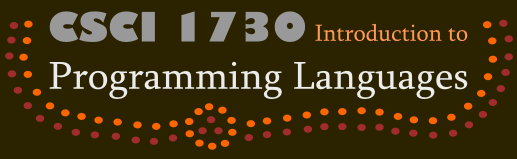You will write a simple survey, the details of which are specified below. Although this program is quite simple, it is representative of the core of much larger Web software (just as the interpreters we write in this course are themselves quite lean, but represent the core of large programming languages).

Your program must have the following features:

1. When users finish the survey, they should see a list containing all of the questions they were asked and their responses. This list should not include any questions which a particular user was not asked.
2. The back button should work properly. If a user answers a question, clicks the back button, then reanswers the question, the later answer should take effect.
3. Window cloning should work properly. If a user fills out the survey to a certain point, then creates a new window (or tab) which is a copy of the current one, then it should be possible to fill out both surveys independently. Furthermore, clicking the back button in one window should not affect any others.

### Survey Specification

1. Do you play World of Warcraft? Answer is yes or no. If yes, go to question 2. If no, end the survey.

2. What level is your highest-level character? Answer is a positive integer. Go to question 3.

3. What is that character's class? Answer is a string. If the answer is "Mage", go to question 4. Otherwise, go to question 5.

4. How many spells does your character know? Answer is a non-negative integer. If the answer to question 2 is 60 or less, go to question 6. Otherwise, end the survey.

5. Why don't you play a mage? Answer is a string. If the answer to question 2 is 60 or less, go to question 6. Otherwise, end the survey.

6. Have you played the expansion? Answer is yes or no. End the survey.

### Web Programming in Racket

You will write your survey system using Racket's Web Server. Use this "Hello, world" template for your program:

```#lang web-server/insta
(require plai/datatype)

(define (start initial-request)
"Hello, world")
```

### Extended Example

The following Web application adds two numbers. Run it and read it to understand how it works.

```#lang web-server/insta
(require plai/datatype)

(define (make-number-page msg)
(lambda (k-url)
`(html
(body
(form ((action ,k-url) (method "post"))
(h2 ,msg)
(input ((type "text") (name "num")))
(input ((type "submit") (name "submit") (value "Submit"))))))))

(define (make-result-page x)
(lambda (k-url)
`(html
(body
"Result: "
,(number->string x)))))

(define (send-and-get msg)
(local ((define req (send/suspend (make-number-page msg)))
(define bindings (request-bindings req)))
(string->number (extract-binding/single 'num bindings))))

(define (start req)
(let ((num1 (send-and-get "first number"))
(num2 (send-and-get "second number")))
(send/suspend (make-result-page (+ num1 num2)))))
```

### Quasi-Quoting

In Racket, it's possible to begin a quoted list with ``` instead of `'`. This is called quasiquoting, as opposed to quoting. Quasiquoting lets you evaluate specific elements of a quasiquoted expression instead of directly returning the expression. To force evaluation of an element in a quasiquoted expression, prefix the element with a comma. For example, to generate the list `'(1 2 3 4)` you may write ````(1 2 ,(+ 0 3) 4)```. Quasiquoting is a convenient way to insert dynamic elements into HTML templates. See the Racket guide to quasiquoting for more information.

### Handin

Just one member of your group should handin the assignment. From the root directory of your web-server, execute:

`/course/cs173/bin/cs173handin pltweb`